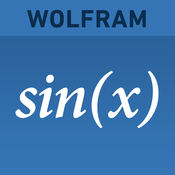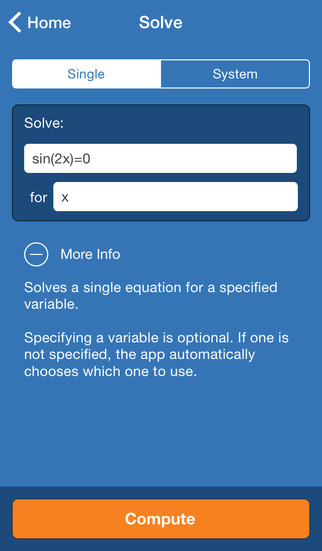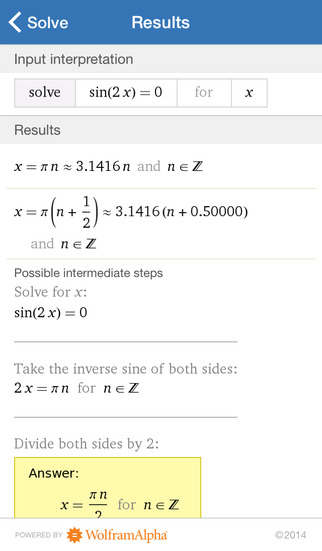3.5

Wolfram Precalculus Course Assistant

• 内容摘要
Taking precalculus? Then you need the Wolfram Precalculus Course Assistant. This definitive app for precalculus—from the world leader in math software—will help you work through your homework problems, ace your tests, and learn calculus concepts. Forget canned examples! The Wolfram Precalculus Course Assistant solves your specific precalculus problems on the fly, including solving equations, vector arithmetic, statistics, and much more.

This app covers the following topics applicable to precalculus and trigonometry:

- Evaluate any numeric expression or substitute a value for a variable
- Solve a single equation or a system of equations
- Plot functions on the x-y plane or draw a parametric or polar plot
- Determine the sine, cosine, and tangent of a specific angle in a right triangle
- Simplify, expand, or factor trigonometric functions
- Find the partial fraction decomposition of an expression
- Calculate the dot product, cross product, and magnitude of two vectors
- Identify the mean, median, mode, and standard deviation of a set of data
- Calculate permutations and combinations

The Wolfram Precalculus Course Assistant is powered by the Wolfram|Alpha computational knowledge engine and is created by Wolfram Research, makers of Mathematica—the world's leading software system for mathematical research and education.

The Wolfram Precalculus Course Assistant draws on the computational power of Wolfram|Alpha's supercomputers over a 3G, 4G, or Wi-Fi connection.

展开↓

• 版本中的新功能

展开↓

• 应用截图••同类热门推荐

••AnkiMobile Flashcards 2.0.48 | 24.30MB
•Toca Kitchen 2 1.2.1 | 84.30MB
••开发商的其他应用

•WolframAlpha 1.7.3 | 30.40MB
••••不可错过安装同步助手后能更便捷的下载此应用• 免费下载游戏和应用
• 快速安装APP
• 便捷管理、优化手机
A连接设备至同步助手，自动安装“Wolfram Precalculus Course Assistant”

安装步骤• 色情内容
• 赌博
• 暴力内容
• 反动内容
• 侵犯版权
• 木马病毒
• 恶意扣费
• 不良插件
• 无法安装或者启动
• 其他